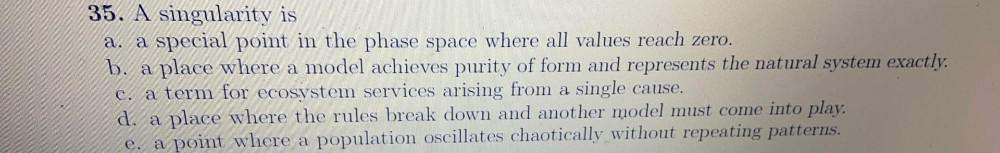Question:

# 35. A singularity is a. a special point in the phase space where all values reach zero. b. a place where a model achieves purity35. A singularity is a. a special point in the phase space where all values reach zero. b. a place where a model achieves purity of form and represents the natural system exactly. c. a term for ecosystem services arising from a single cause. d. a place where the rules break down and another model must come into play. e. a point where a population oscillates chaotically without repeating patterns. a